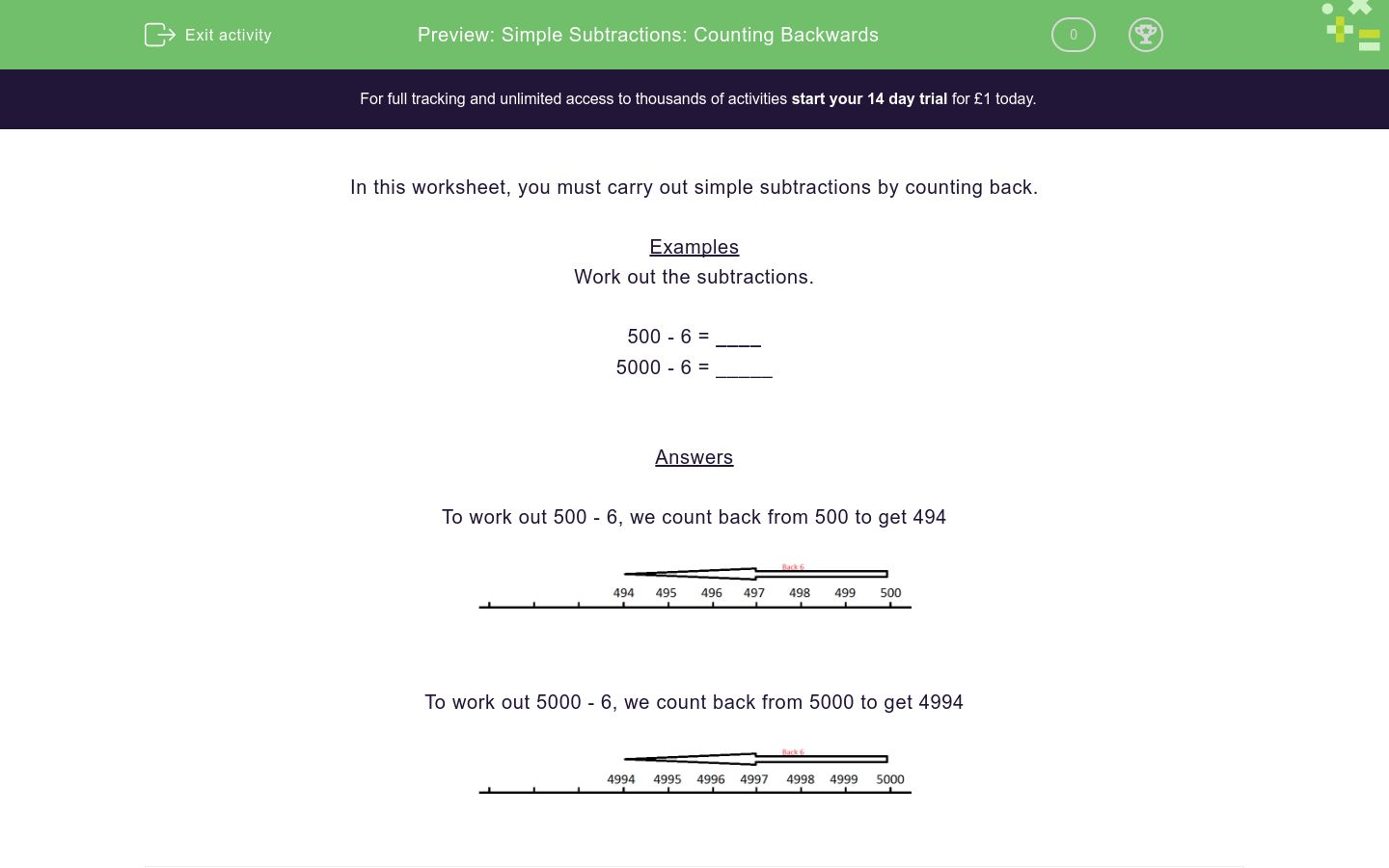# Simple Subtractions: Counting Backwards

In this worksheet, students carry out simple subtractions by counting back on a number line.Key stage:  KS 2

Curriculum topic:   Number: Addition and Subtraction

Curriculum subtopic:   Add/Subtract up to Four-Digit Numbers

Difficulty level:### QUESTION 1 of 10

In this worksheet, you must carry out simple subtractions by counting back.

Examples

Work out the subtractions.

500 - 6 = ____

5000 - 6 = _____

To work out 500 - 6, we count back from 500 to get 494To work out 5000 - 6, we count back from 5000 to get 4994Work out the subtraction.

600 - 8 = __

Work out the subtraction.

700 - 3 = __

Work out the subtraction.

800 - 3 = __

Work out the subtraction.

700 - 6 = __

Work out the subtraction.

5000 - 9 = __

Work out the subtraction.

6000 - 8 = __

Work out the subtraction.

9000 - 9 = __

Work out the subtraction.

6000 - 2 = __

Work out the subtraction.

4000 - 10 = _____

Work out the subtraction.

400 - 10 = ____

• Question 1

Work out the subtraction.

600 - 8 = __

592
• Question 2

Work out the subtraction.

700 - 3 = __

697
• Question 3

Work out the subtraction.

800 - 3 = __

797
• Question 4

Work out the subtraction.

700 - 6 = __

694
• Question 5

Work out the subtraction.

5000 - 9 = __

4991
• Question 6

Work out the subtraction.

6000 - 8 = __

5992
• Question 7

Work out the subtraction.

9000 - 9 = __

8991
• Question 8

Work out the subtraction.

6000 - 2 = __

5998
• Question 9

Work out the subtraction.

4000 - 10 = _____

3990
• Question 10

Work out the subtraction.

400 - 10 = ____

390
---- OR ----

Sign up for a £1 trial so you can track and measure your child's progress on this activity.

### What is EdPlace?

We're your National Curriculum aligned online education content provider helping each child succeed in English, maths and science from year 1 to GCSE. With an EdPlace account you’ll be able to track and measure progress, helping each child achieve their best. We build confidence and attainment by personalising each child’s learning at a level that suits them.

Get started